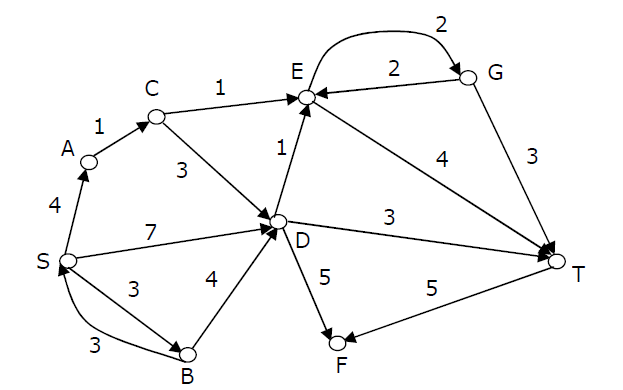# Algorithms | Graph Shortest Paths | Question 14

Consider the directed graph shown in the figure below. There are multiple shortest paths between vertices S and T. Which one will be reported by Dijstra?s shortest path algorithm? Assume that, in any iteration, the shortest path to a vertex v is updated only when a strictly shorter path to v is discovered.(A) SDT
(B) SBDT
(C) SACDT
(D) SACET

Explanation: See Dijkstra’s shortest path algorithm

When the algorithm reaches vertex ‘C’, the distance values of ‘D’ and ‘E’ would be 7 and 6 respectively. So the next picked vertex would be ‘E’

Quiz of this Question

My Personal Notes arrow_drop_up

Article Tags :

Be the First to upvote.

Please write to us at contribute@geeksforgeeks.org to report any issue with the above content.